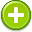#weighted 中文解釋 wordnet sense Collocation Usage
Verb
/wāt/,Font size:weighted, past participle; weighted, past tense; weighting, present participle; weights, 3rd person singular present;
1. Hold (something) down by placing a heavy object on top of it
• - a mug half filled with coffee weighted down a stack of papers
2. Make (something) heavier by attaching a heavy object to it, esp. so as to make it stay in place
• - the jugs were covered with muslin veils weighted with colored beads
3. Attach importance or value to
• - speaking, reading, and writing should be weighted equally in the assessment
4. Be planned or arranged so as to put a specified person, group, or factor in a position of advantage or disadvantage
• - the balance of power is weighted in favor of the government
5. Multiply the components of (an average) by factors to take account of their importance

6. Assign a handicap weight to (a horse)

7. Treat (a fabric) with a substance to make it seem thicker and heavier

2. adjusted to reflect value or proportion; "votes weighted according to the size of constituencies"; "a law weighted in favor of landlords"; "a weighted average"
3. (weighting) weight: (statistics) a coefficient assigned to elements of a frequency distribution in order to represent their relative importance
4. A weight function is a mathematical device used when performing a sum, integral, or average in order to give some elements more "weight" or influence on the result than other elements in the same set. ...
5. A-weighting is the most commonly used of a family of curves defined in the International standard IEC 61672:2003 and various national standards relating to the measurement of sound pressure level (as opposed to actual sound pressure). The others are B, C, D and now Z weightings (see below).
6. (Weighting) The process of weighting involves emphasising some aspects of a phenomenon, or of a set of data — giving them 'more weight' in the final effect or result. It is analogous to the practice of adding extra weight to one side of a pair of scales to favour a buyer or seller.
7. Having weights on it; biased, so as to favour one party
8. (weighting) weights
9. (Weighting) a method of rating the degree of importance of a factor or variable.
10. Weighting is when the responses from some (or all) sub-groups are assigned a statistical weight to reflect the importance of the sub-group in the population of interest.
11. (A-weighting) A frequency-response adjustment of a sound-level meter that makes its reading conform, very roughly, to human response.
12. (Weighting) A process used to ensure that statistics produced from a sample are representative of the population from which the sample was drawn. Most large surveys include weights and descriptive statistics produced from such surveys should be based on weighted data.
13. (A-WEIGHTING) A measure of sound pressure level designed to reflect the response of the human ear, which does not respond equally to all frequencies. ...
14. (A-Weighting) Measurement based roughly on the uneven frequency sensitivity of the human ear. The influences of low and high frequencies are reduced in comparison to midrange frequencies because people are most sensitive to midrange sounds.
15. (A-weighting) A standard compensation (weighting) curve sometimes applied to audio measurements to account for the ear's response to low-level sound. Frequently applied to measurements of the residual noise levels of wireless microphone systems and other electronic devices. ("A-weighted noise.")
16. (Weighting) The proportion of a share or asset class in a portfolio of a fund compared with the proportion in an index.
17. As in, "weighting the rope." Any time the rope takes the weight of the climber. This can happen during a minor fall, a whipper (long fall), or simply by resting while hanging on the belay rope (see also hangdogging.)
18. (Weighting) the amount of weight or relative importance placed on a certain type of investment for ethical or financial reasons, which takes into account quantifiable and unquantifiable importance.
19. (weighting) Mathematically assigning to any given test item that proportion of the total score it will determine.
20. (A WEIGHTING) An electronic filtering system in a sound meter that allows meter to largely ignore lower frequency sounds in a similar fashion to the way our ears do.
21. (A-WEIGHTING) A frequency weighting network used to account for changes in sensitivity as a function of frequency (See Section 3.1.3.4.2).
22. (WEIGHTING) Component series are equally weighted in the aggregation process into a country CLI. On the other hand, GDP-PPP weights are used to estimate the CLIs for groups of countries, i.e. zone.
23. (WEIGHTING) Each day in the Peoplemeter sample the distribution of homes and people is checked against Statistics NZ Census data to ensure the correct proportions are in place. ...
24. (WEIGHTING) Prescribed frequency filtering provided in a sound level meter.
25. (Weighting) (1) For evaluation of customer lists, a means of applying values to the RF\$UISM data for each cell. (For larger lists this is better done by a computer regression analysis); (2) for merge/purge, a means of applying a form of mathematical analysis to each component for unduplicating.